# Computation and Problem-Solving Strategies

One of the most difficult areas identified by after-school staff is helping students with math. We include some tips on helping students who are being taught computation with some newer and more traditional strategies. We also provide a model for helping students think through word problems that has emerged from high-achieving East Asian countries.

### A. Strategies that use and build number sense

Addition and subtraction: Working with ten(s)
Help students use numbers that are easy to work with. Get the very young to work with 10. It is easy to add numbers that end in zero in your head. Students should become very comfortable with all the combinations that make 10!

 Problem The mathematical thinking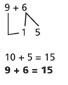To make 9 into 10 you need 1 more so think of 6 as 1 & 5.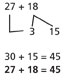To make 27 into 30 you need 3. Split 18 into 3 & 15.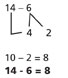To subtract from 10, first take away 4 ones. See 6 as 4 & 2.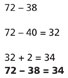It is easy to subtract numbers ending in zero. Subtract 40.But we subtracted 2 too many. We must put them back now.

### B. Multiplication and division

Children need to understand that multiplying is counting by groups of things, each group having the same quantity. Help them see how the tables are built one group at a time. Some solution strategies that help students understand the multiplication algorithm are:

1. Build an array to show the meaning of multiplication.

(4 rows of 12)

 4 x 12 Mathematical thinking OOOOOOOOOOOOOOOOOOOOOOOOOOOOOOOOOOOOOOOOOOOOOOOO 1 row has 122 rows have 243 rows have 364 rows have 48

2. T-tables. These numbers can be recorded in a t-table that gives meaning to the multiplication tables.

 Rows Total 1 12 2 24 3 36 4 48

Later, students can begin reasoning with such tables and not have to write every number.

 12 boxes with 18 items per box No. Pkgs. No. Items Reasoning 1 18 Given 2 36 Add another 18 4 72 Double amount for 2 boxes 6 108 Add amounts in 2 and 4 boxes

3. Area models

These models are more abstract and can allow students to see partial products and can eventually be linked to the traditional algorithm.

Begin by building an array model with base-10 blocks. Students must know how to break numbers apart by place value to do this. Here is an illustration for 24 x 45. (You would start with something simpler, such as 8 x 12.)

24 is split into 20 and 4. 45 is split into 40 and 5.

 X 40 5 20 100 100 100 100 10 10 10 10 10 100 100 100 100 10 10 10 10 10 4 10 10 10 10 1 1 1 1 1 10 10 10 10 1 1 1 1 1 10 10 10 10 1 1 1 1 1 10 10 10 10 1 1 1 1 1

There are eight 100 blocks or 8 x 100 = 800. There are 26 blocks of 10 or 26 x 10 = 260. There are 20 blocks of 1 or 20 x 1 = 20.

800
260
+ 20
1080

Eventually students chunk numbers and draw four large blocks. This mirrors the fundamental distributive property.

 40 + 5 20+4 20x40800 20x5100 4x40160 4x520

800 + 160 = 960 100 + 20 = 120
960 + 120 = 1,080

Then you can connect the numbers from the area model to a multiplication problem. Keep the full quantities visible at first. It is 20 times 40 not 2 times 4. When this level is clear, do the same thing but show how you can start the calculation in ones place. Continue to record the full quantities. Finally, when that routine is comfortable, show how the traditional algorithm is another way of doing the same thing. This time not all the partial products are recorded. There is some remembering in the head that you have more tens. What is very tricky is that they cannot be added to the tens place number until that multiplication has been done. Students who have difficulty “moving over” or remembering when to add in the regrouped figures should be allowed to write the complete numbers as partial products.

4. Division

The language used for division can be an impediment to students understanding what division is all about. For example, to say “9 goes into 81, 9 times” is difficult to visualize. What does that mean? Why does it “go in” so many times? Ask instead, how many groups of 9 are there in 81?

Before students are forced to think abstractly, help them understand what these numbers stand for. Since division is the inverse of multiplication, we are still dealing with groups. In the problem 3,528 divided by 24, they are finding how many groups of 24 there are in 3,528 or how many groups of size 24 could be made. Could there be 100? Certainly. 100 x 24 is 2,400. That leaves 1,128. Then some students might recognize there are at least 20 more. Others might stick with thinking 10 more at a time.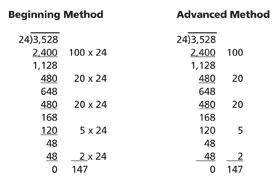As they work through the problem, help them understand what has been accounted for so far and how many more are still left to put into groups.